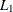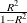# The FASTCLUS Procedure

### Output Data Sets

#### OUT= Data Set

The OUT= data set contains the following:

• the original variables

• a new variable indicating the cluster assignment status of each observation. The value will be less than the permitted number of clusters (see the MAXCLUSTERS= option) if the procedure detects fewer clusters than the maximum. A positive value indicates the cluster to which the observation was assigned. A negative value indicates that the observation was not assigned to a cluster (see the STRICT option), and the absolute value indicates the cluster to which the observation would have been assigned. If the value is missing, the observation cannot be assigned to any cluster. You can specify the variable name with the CLUSTER= option. The default name is CLUSTER.

• a new variable, DISTANCE, giving the distance from the observation to its cluster seed

If you specify the IMPUTE option, the OUT= data set also contains a new variable, _IMPUTE_, giving the number of imputed values in each observation.

#### OUTSEED= Data Set

The OUTSEED= data set contains one observation for each cluster. The variables are as follows:

• the BY variables, if any

• a new variable giving the cluster number. You can specify the variable name with the CLUSTER= option. The default name is CLUSTER.

• either the FREQ variable or a new variable called _FREQ_ giving the number of observations in the cluster

• the WEIGHT variable, if any

• a new variable, _RMSSTD_, giving the root mean squared standard deviation for the cluster. See Chapter 31: The CLUSTER Procedure, for details.

• a new variable, _RADIUS_, giving the maximum distance between any observation in the cluster and the cluster seed

• a new variable, _GAP_, containing the distance between the current cluster mean and the nearest other cluster mean. The value is the centroid distance given in the output.

• a new variable, _NEAR_, specifying the cluster number of the nearest cluster

• the VAR variables giving the cluster means

If you specify the LEAST=p option with a value other than 2, the _RMSSTD_ variable is replaced by the _SCALE_ variable, which contains the pooled scale estimate analogous to the root mean squared standard deviation but based on pth-power deviations instead of squared deviations:

LEAST=1

mean absolute deviation

LEAST=p

root mean p-th-power absolute deviation

LEAST=MAX

maximum absolute deviation

If you specify the OUTITER option, there is one set of observations in the OUTSEED= data set for each pass through the data set (that is, one set for initial seeds, one for each iteration, and one for the final clusters). Also, several additional variables appear:

_ITER_

is the iteration number. For the initial seeds, the value is 0. For the final cluster means or centers, the _ITER_ variable is one greater than the last iteration reported in the iteration history.

_CRIT_

is the clustering criterion as described under the LEAST= option.

_CHANGE_

is the maximum over clusters of the relative change in the cluster seed from the previous iteration. The relative change in a cluster seed is the distance between the old seed and the new seed divided by a scaling factor. If you do not specify the LEAST= option, the scaling factor is the minimum distance between the initial seeds. If you specify the LEAST= option, the scaling factor is anscale estimate and is recomputed on each iteration.

_HOMPAR_

is the value of the homotopy parameter. This variable appears only for LEAST=p with 1 < p < 2.

_BINSIZ_

is the maximum bin size used for estimating medians. This variable appears only for LEAST=1.

If you specify the OUTITER option, the variables _SCALE_ or _RMSSTD_, _RADIUS_, _NEAR_, and _GAP_ have missing values except for the last pass.

You can use the OUTSEED= data set as a SEED= input data set for a subsequent analysis.

#### OUTSTAT= Data Set

The variables in the OUTSTAT= data set are as follows:

• BY variables, if any

• a new character variable, _TYPE_, specifying the type of statistic given by other variables (see Table 36.2 and Table 36.3)

• a new numeric variable giving the cluster number. You can specify the variable name with the CLUSTER= option. The default name is CLUSTER.

• a new numeric variable, OVER_ALL, containing statistics that apply over all of the VAR variables

• the VAR variables giving statistics for particular variables

The values of _TYPE_ for all LEAST= options are given in Table 36.2.

Table 36.2: _TYPE_

_TYPE_

Contents of VAR Variables

Contents of OVER_ALL

INITIAL

Initial seeds

Missing

CRITERION

Missing

Optimization criterion (see the LEAST= option); this value is displayed just before the Cluster Summary table.

CENTER

Cluster centers (see the LEAST= option)

Missing

SEED

Cluster seeds: additional information used for imputation

DISPERSION

Dispersion estimates for each cluster (see the LEAST= option); these values are displayed in a separate row with title depending on the LEAST= option

Dispersion estimates pooled over variables (see the LEAST= option); these values are displayed in the Cluster Summary table with label depending on the LEAST= option.

FREQ

Frequency of each cluster omitting observations with missing values for the VAR variable; these values are not displayed

Frequency of each cluster based on all observations with any nonmissing value; these values are displayed in the Cluster Summary table.

WEIGHT

Sum of weights for each cluster omitting observations with missing values for the VAR variable; these values are not displayed

Sum of weights for each cluster based on all observations with any nonmissing value; these values are displayed in the Cluster Summary table.

Observations with _TYPE_=’WEIGHT’ are included only if you specify the WEIGHT statement.

The _TYPE_ values included only for least squares clustering are given Table 36.3. Least squares clustering is obtained by omitting the LEAST= option or by specifying LEAST=2.

Table 36.3: _TYPE_

_TYPE_

Contents of VAR Variables

Contents of OVER_ALL

MEAN

Mean for the total sample; this is not displayed

Missing

STD

Standard deviation for the total sample; labeled Total STD in the output

Standard deviation pooled over all the VAR variables; labeled Total STD in the output

WITHIN_STD

Pooled within-cluster standard
deviation

Within cluster standard deviation pooled over clusters and all the VAR variables

RSQ

R square for predicting the variable from the clusters; labeled R-Squared in the output

R square pooled over all the VAR variables; labeled R-Squared in the output

RSQ_RATIO; labeled RSQ/(1-RSQ) in the output; labeled RSQ/(1-RSQ) in the output

PSEUDO_F

Missing

Pseudo F statistic

ESRQ

Missing

Approximate expected value of R square under the null hypothesis of a single uniform cluster

CCC

Missing

Cubic clustering criterion